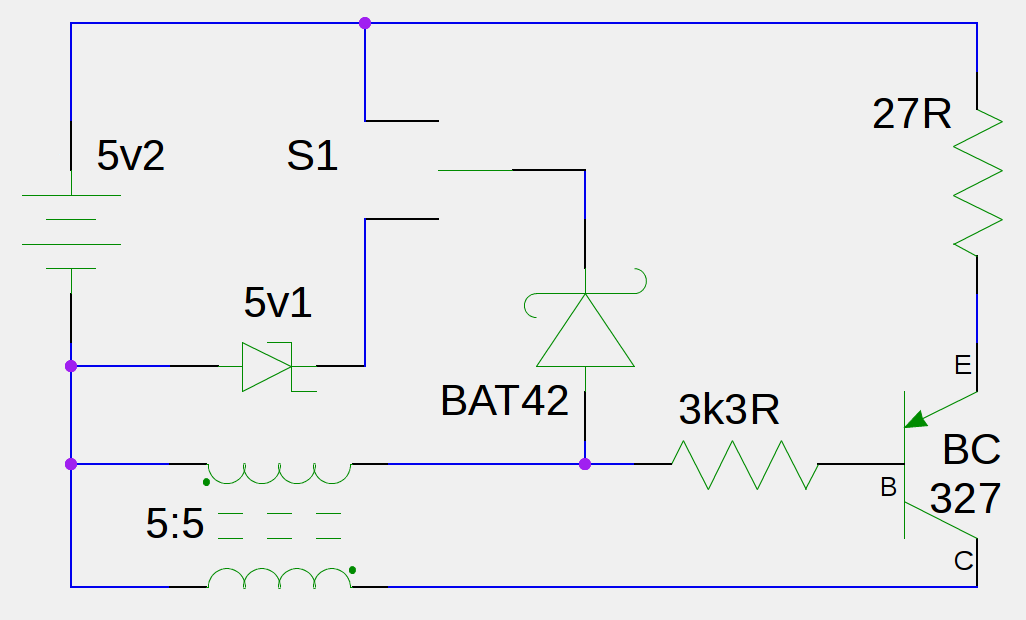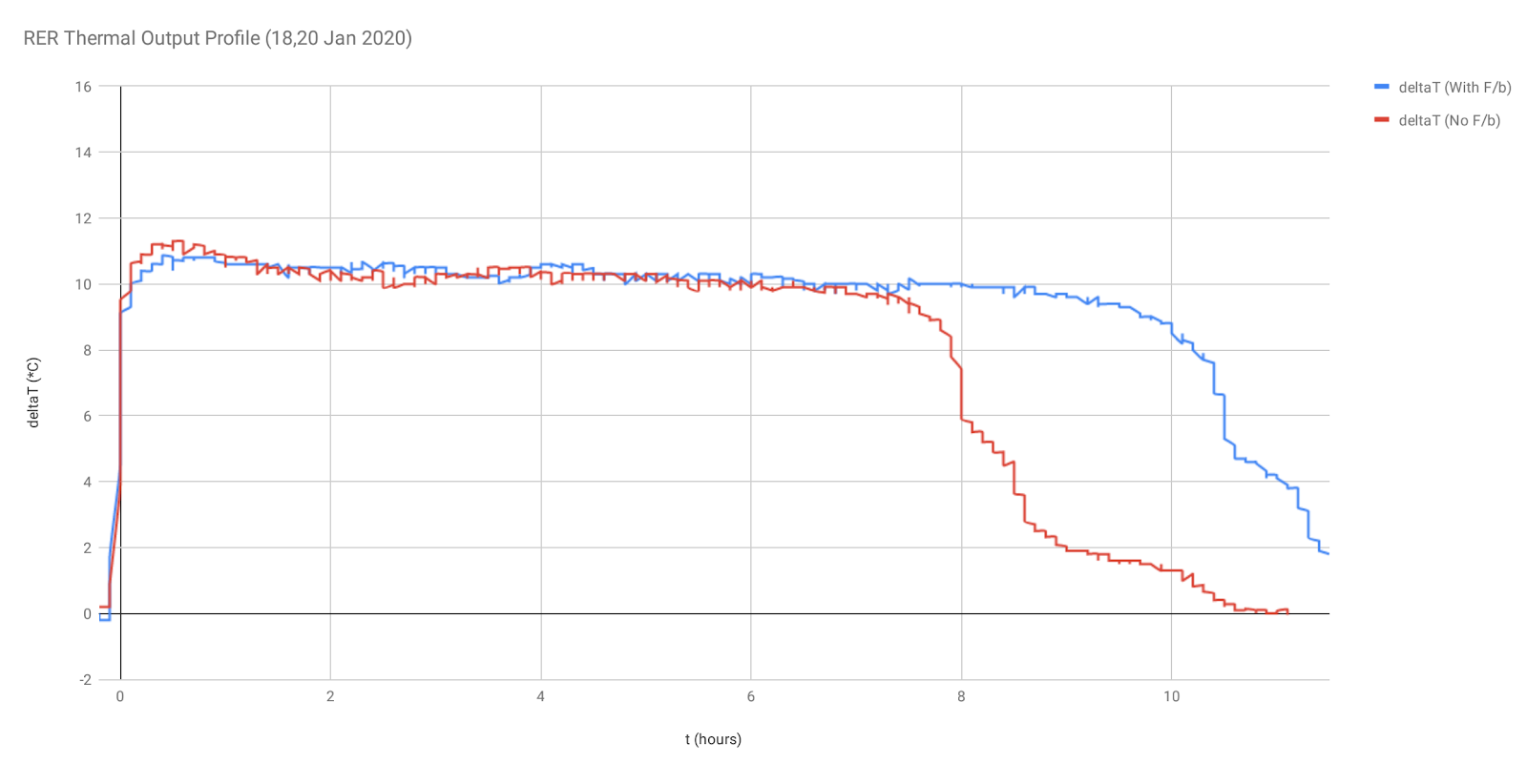## Saturday 1 February 2020

### Heat Data Confirms Battery Energy Conversion > 100%

Background
Previous tests provided results using measurements of duration, battery terminal voltage, and load resistances.  Those results indicated that the total initial energy stored in the test circuit's rechargeable battery could be effectively 'recycled' by feeding a proportion of the input energy back to the battery from temporary storage within the circuit

Energy-to-work conversion ratios in the range 170-200% were observed

The circuit operates with all the usual inefficiencies but the duration of its operation becomes extended due to the real-time recharging of its supply battery

Thermal Profile testing
These latest tests confirm the ability to recycle some of the original energy, by measuring the thermal activity of the total system:
-  the reference test measures the temperature at the load resistor, against time, when all the input energy is dissipated directly within the circuit (results labelled as 'No F/b');
- the active test measures the temperature at the load resistor, against time, for the arrangement where some energy is used to partially recharge the supply battery (results labelled as 'With F/b')

The temperature of the load resistor is related to the current it carries - the value of the heat energy dissipated by the resistor is given by the product of the resistance with the square of the current and with time

The circuit has been arranged in such a way that the total supply current for the circuit is the same for both the reference test and the active test.  If the results show that the same temperature is achieved at the load resistor for the duration of both tests, then the comparative duration values will indicate whether or not the circuit arrangement with energy feedback to the battery (See Fig. 1) can effectively operate for longer (ie. produce more work) than the circuit which just dissipates all the energy supplied from the battery into the circuit in one passFig. 1 - Ringwood Energy Recycler Block Diagram

Test procedure
The circuit has been simplified and re-arranged to provide for measurement of temperature on a single 27 ohm load resistor which is in the input path of the total current supplied to the circuit (See Fig 2.). The supply battery voltage has been increased to 5.2V (nominal) in order to improve the significance of the temperature rise compared with the ambient.  The temperature values, deltaT, presented on the graph represent the difference between the temperature probe, monitoring the load, and the sensor monitoring the ambient temperature

The circuit is tested in two configurations:
1. (Reference test) the output pulse from the BAT42 diode is connected to the cathode of a 5v1 zener diode, to dissipate the pulse energy within the circuit;
2. (Active test) the output pulse from the BAT42 diode is connected to the +ve terminal of the battery, where it provides a partial recharge with the pulse energy

The switch S1 shown in Fig 2. provides these 2 test arrangements, a) and b)Fig. 2 - Test circuit used to obtain thermal output profile

The circuit and battery are completely enclosed within a metal container, which is lined with a layer of heat-insulation.  A thermistor temperature probe is fixed to the 27 ohm load resistor;
A second thermistor sensor records ambient temperature (within the datalogger interface)

Results
The numerical results, obtained from the data spreadsheet calculations, show that for a full discharge of energy from the battery in each case:-
1. (Reference test)
sustained an average deltaT of 9.92 degC for a total of 8.4 hours
1. (Active test)
sustained an average deltaT of 9.98 degC for a total of 10.6 hours

(The test durations are measured between mid-point values of the rising and falling edges of each temperature profile; the temperature profile for each test has been aligned then at the selected point for that test, to provide a direct comparison of their respective durations)

These results are presented graphically in Fig 3:Fig. 3 - Thermal Output Profile results

A separate discharge test of the same battery, using a 68 ohm resistor, produced a value of 10650 Joules for the total energy stored by the battery when fully charged;  using this value, the average current drawn by both the reference and the active test circuits is calculated to be approx 70mA

Conclusions
The results confirm that both the reference test and the active test are operating with the same average supply current, as evidenced by close agreement of the average temperatures measured at the load resistor in the supply path

Since the active test duration is longer than the duration of the reference test, the circuit with feedback of energy to its supply battery is shown to have increased the total amount of work converted from that battery by a factor of  (10.6 / 8.4) = 1.26

These results confirm the results of previous testing (mentioned above);  the initial energy obtained and converted from the battery (10650 Joules) and temporarily stored within particular circuit components can then be used to partially recharge the supply battery in real-time and extend the total amount of work which is converted by the whole system (13450 Joules)

The example arrangement tested here effectively extended the total amount of work converted from the supply battery to 126%

## Thursday 29 November 2018

### Observing a 170% conversion of battery energy into work

The basis of the Ringwood Energy Recycler circuit used in these tests is not particularly unusual - it's a simple blocking oscillator and it has a work-efficiency of approximately 85% (quantifiable-work performed for total energy supplied)

#### Fig. 1 - Circuit with current feedback to supply battery

Unquantified losses include resistive heat-loss in the switching transistor & diode, coil windings, and transistor biasing

The switching circuit operates at approx 67 kHz, in 2 half-cycles: a `Drain` step, followed by a `Recharge` step;   DC output to the LEDs is provided by a 100uF buffer capacitor

#### Fig. 2 - Current paths in the system

The battery capacity can be characterised by discharging it using a resistor with a known value, over the terminal-voltage range, approximately 4.2V to 3.45V

Using a battery with 3 NiMH cells of 750mAh rated capacity, a fully charged battery supplied a 268 ohm (measured) resistor with an average power of 14.4mA x 3.86V for 42.5 hours, converting a total of 8504 Joules of energy

When the discharge resistor is replaced with the preliminary circuit (ie. the feedback diode is connected to a spare battery which is not the supply battery, but similar construction) the quantifiable work consists of two parts:-
- converting 3105 Joules at the output, to illuminate some LEDs
- recharging the spare battery by using 4238 Joules of energy which is being temporarily stored in the circuit as a by-product of the oscillator operation

The circuit provides this quantifiable work for a total of 30.5 hours, drawing an average of 20.1mA at 3.86V to draw a total of 8603 Joules (approximately matching the total energy, 8504 Joules, converted by the nominal 270 ohm resistor)

In this mode, the circuit draws the total energy available and converts this to 7343 Joules of quantifiable work, giving a quantifiable-work efficiency of 85%

When the feedback diode is re-connected to the supply mode (see Fig. 1), the circuit will operate for almost twice the duration of the conventional, non-feedback mode - the circuit now operates for a total of 60.5 hours, whilst drawing the same real average power, 20.1mA at 3.86V,  therefore the total energy now drawn by the circuit is 16898 Joules

The 'With-feedback' circuit operating duration has been increased to almost twice that of the original circuit (60.5 hours compared to 30.5) with the loads remaining the same values

[The circuit can operate at the same power drain for longer because it is constantly re-charging its supply with a proportion of the energy which has been input - the actual supply current drained is pulsed, with an average of 20.1mA, whilst 10mA av. is recharged back into the battery, being interleaved each cycle similar to time-division-multiplexed operation (see Figs. 2 & 3), resulting in a 'virtual' current drain from the battery of (20.1mA - 10mA) = 10.1mA av.]

#### Fig. 3 - current pulses in supply connection (blue trace)

The efficiency of the oscillator circuit compared to its total energy drawn hasn't changed essentially:
the quantifiable work has increased to 14566 Joules (approx 6200J driving LEDs and approx 8400J recharging battery), and these values give an efficiency of 14566 / 16898 = 86%

The overall system efficiency for quantifiable work from the original energy in the battery, however, is now 14566 / 8504 = 170%

The total system efficiency for conversion of energy in the whole system (battery + circuit) now becomes: 16898  / 8504 = 199%

The system is recycling its input energy by a factor of 1.99

The 'feedback to supply' mode has extended the duration for circuit operation and enabled the amount of useful energy available for the LEDs to be approximately doubled compared to the 'no feedback' arrangement (see Fig. 4 below)

#### (Blue traces: Battery discharge profiles; Red traces: LED drive levels)

Although the total work converted by the circuit LEDs, on their own, remains less than the original supply of energy, and the energy converted by those circuit LEDs matches that of a passive resistor-driven LED arrangement (6159J vs. 6169J), the 'With-Feedback' current arrangement still enables the circuit to produce a light output level equivalent to the passive DC-drive arrangement (146 Ft-candles vs 140 approx. for  DC drive, both measured at average Vsupply of 3.86V) but for approximately 34% longer duration (60.5 hours vs. 45 hours, as shown below in Fig.5)

#### (Blue traces: Battery discharge profiles;   Red traces: LED drive levels)

This resulting system behaviour provides a worthwhile gain, which has been enabled by the 200% conversion to total system effect (16898J) of the original store of energy in the battery (8504J)